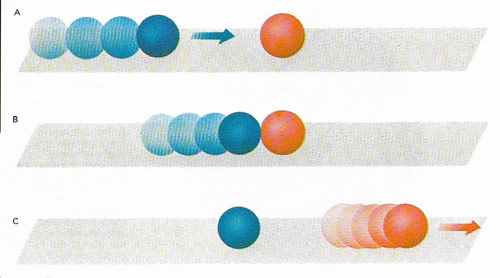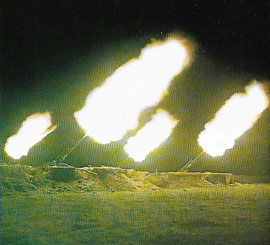A# conservation lawFigure 1. A stationary ball is hit by another of similar mass moving toward it. Before the collision (A) the moving ball has a certain momentum (equal to the product of its mass and its velocity). At the moment of impact (B), this momentum is transferred to the stationary ball, which had no momentum. As a result, the stationary ball moves while the ball that was moving formerly becomes stationary (C). In all such collisions momentum is conserved – after impact the momentum of the second ball equals that of the first.

A conservation law is a law which states that some property of a closed system is unaltered by changes in that system. Among the most important are the law of conservation of mass and energy and the law of conservation of momentum.

## Law of conservation of mass and energy

The law of conservation of mass and energy is a principle, resulting from Einstein's special theory of relativity, which combines the separate laws of conservation of mass and of energy. The law of conservation of mass (or matter) states that in any system matter cannot be created or destroyed and the law of conservation of energy states that in any system energy cannot be created or destroyed. These laws are now seen to be approximations which can only be applied to systems not involving nuclear reactions or speeds approaching the speed of light. The general principle of the conservation of mass and energy, which is of universal applicability, is based upon the mass-energy relationship.

## Law of conservation of momentumFigure 2. A heavy gun recoils when firing and an equal and opposite reaction propels the shell forwards. The principle of conservation of momentum states that the total momentum before and after is zero but the shell is given its forward velocity because it is lighter than the gun. The chemical energy stored in the propellant explosive charge is transformed into the kinetic energies of gun and shell.

Conservation of momentum is a fundamental law of motion, equivalent to Newton's laws: in a system of bodies, the vector sum of all momenta cannot change due to any internal interactions. See Figure 1 and 2.